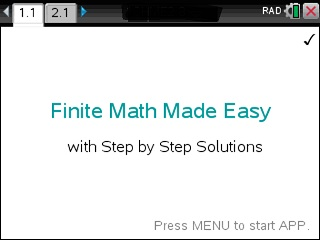## App Purchase

Enter the last 8 digits of your 27-digit TI-Nspire's Product ID.

Located under 5:Settings → 4:Status → About ID may look like: 1008000007206E210B0BD92F455 HELP. If this was your ID you would only type in BD92F455.

At the end of the PayPal checkout, you will be sent an email containing your app. Read Installation InstructionsPrice:\$49.95

## Description

• The most comprehensive Finite Math APP for calculators.
• Offers the same feature as Discrete Math Made Easy
• Step by Step Analysis of..
• Linear Programming
• Marginal Analysis, Demand Analysis
• Markoff Chains
• Simplex Algorithm
• Probabilities
• Combinatorics
• Functions
• Vector and Matrices
• Solve Equations
• Logic with Set Theory, Truth Tables
• Users have boosted their Finite Math knowledge.
• Ideal for quick review and homework check in Finite Math classes.
• Easy to use. Just plug in the equation and the correct answer shows.

## FUNCTIONALITY & MENU ITEMS OF APP :

### LOGIC

• Read Set Theory: 10 Facts
• Read Set Theory: 9 Laws

### ALGEBRA

• Solve any Equation or Inequality
• Solve 2x2 system - Step by Step
• Simplify & Evaluate
• Factor
• Powers
• Expand/Distribute
• Find GCD & LCM
• Find Common Denominator
• Find Proper Fractions
• Solve Proportion (Ratio) Problems
• Absolute and Percent Change

### FUNCTIONS

• All-in-one-Function Explorer
• Evaluate Function
• Find Domain of f(x)
• Find Range of f(x)
• Intersection of 2 Functions
• Find f+g
• Composition of 2 Functions f(g(x))
• Find [f(x+h)-f(x)]/h
• Find Inverse function
• Interval Notation

### POLYNOMIALS & SEQUENCES

• All-in-one-Polynomial Explorer
• Find Degree
• Find Roots
• Polynomial Division & Remainder
• Synthetic Division
• Explicit Sequence & Partial Sum
• Recursive Sequence & Partial Sum
• Sequence Formula Finder
• Geometric Sequence & Series
• Arithmetic Sequence

### POINTS & LINES

• Find Slope
• Find y=mx+b
• Find Point Slope & y=mx+b given Pt & Slope
• Check if 2 Lines are Parallel or Perpendicular
• Find Parallel and Perpendicular Lines
• Read: Absolute Value Function |x|

### QUADRATIC EQUATIONS & COMPLEX NUMBERS

• Complete the Square
• Complete the Square to find Zeros
• Complete the Square to find Vertex
• One Complex Number : All-in-one-Explorer
• Two Complex Numbers : All-in-one-Explorer

### COMBINATORICS

• n1*n2*n3*.. = Multiplication Principle
• n! = Number of Rearrangements
• nPr = Number of ways to rearrange r given n
• nCr = Number of ways to pick r given n
• n^r = Number of ways to rearrange n items repeated r times
• (n+r-1)!/((n-1)!*r!) = Select r given n w. repeatition
• Binomial Coefficients & Pascal Triangle

### PROBABILITY & EXPECTED VALUE

• Odds <-> Probability
• Conditional Probability
• Expected Value: Find mu and s
• P(A or B)=P(A)+P(B)-P(A and B)
• Check if A and B are dependent or disjoint
• P(A or B or C)

### VECTORS

• All-in-one-1-Vector Explorer
• Norm
• All-in-one-2-Vector Explorer
• Test of Orthogonality & Angle between A and B
• Projection A to B
• Test of (In)Dependence
• Cross Product finds perpendicular Vector
• Find Orthogonal 3D Vector

### GAME THEORY

• Read Symmetric vs Non-Symmetric Games
• Read Cooperative vs Non-Cooperative Games
• Read Simultaneous vs Non-Simultaneous Games
• Read Symmetric vs Non-Symmetric Games
• Read Perfect vs Imperfect Information

### MARKOV CHAINS & STOCHASTIC MATRICES

• Stochastic and Regular Stochastic Matrix
• Probability Vector
• Terminal State & Fixed Probability Vector
• Find A^n
• nth State of a Markov Chain: p0*A^n

### MATRICES

• All in one Matrix A Explorer
• Two Matrices: A+B
• Find the Inverse of A
• Find the Determinant of A
• Simplex Algorithm
• Leontief Model
• Row Echolon(A)
• Reverse Row Echolon(A) - STEPS
• Solve System of Equations A*X=B
• Cramer Rule to solve A*X=B

### LINEAR PROGRAMMING

• Find Maximum
• Find Mimimum
• Simplex Algorithm

• Marginal Profit
• Marginal Revenue
• Demand Analysis
• Simplex Algorithm
• Find Equilibrium of Supply & Demand